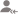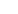truePost a LessonSujoy D.

Tutor

SI= PRT/100 =40,000*7*72/1200. By Solving It. 16,800 RS. Amount = Si + Principal =16800+40000 = Rs. 56,800.
Dislike BookmarkAnurag Singh

Tutor

Rs. 1200.
Dislike BookmarkKumar P.

\$ 459.60
Dislike Bookmark

If you need Rs. 10,000 to pay for your first year of graduate school in 3 years and you get an interest... read more
If you need Rs. 10,000 to pay for your first year of graduate school in 3 years and you get an interest rate of 9%, how much must you invest each of the next three years? read lessKundan

Maths Wizard

This problem is based on installment. Please tell the rate of interest charged is SI or CI.
Dislike Bookmark

If 6 years ago you invested Rs. 500 and received an interest rate of 4% (compounded monthly), how much... read more
If 6 years ago you invested Rs. 500 and received an interest rate of 4% (compounded monthly), how much would you now have? read lessVeera Krishna

Trainer

Rate = 4%= 0.04 Amount = 500 since compounded monthly = no of years x 12=6 x 12 = 72 total amount = 500 x(1+0.04)^72 Rs. 8421.131.
Dislike Bookmark

How many years would it take you to have Rs. 2,500 if you saved Rs. 100 each month at 15%?Ankit

JEE/Board Maths Tutor

Principle for a month=Rs.100 Amount=SI+P= P(1+RT/100)=100(1+0.15T) =>2500=100(1+0.15T) =>25=1+0.15T =>24=0.15T =>T=160 months=13.3 yrs
Dislike Bookmark

To have Rs. 6000 in 7 years what interest rate would you need if you saved \$200 every quarter?Jitender Saini

Physics Expert

1 Dollar = 68.67, Now amount of one year = 200*4*68.67= Rs. 54936, You have interest= 6000, Time =7 yr, Now rate = 6000*100/54936*7= 1.5603 ans.
Dislike Bookmark

How long will it take to repay a loan of Rs. 150, if I pay \$1 per week and the rate on my loan is 4%?Ankit

JEE/Board Maths Tutor

210 weeks.
Dislike Bookmark

Suppose you have the opportunity to make an investment expects to pay investors Rs. 7,000 per year for... read more
Suppose you have the opportunity to make an investment expects to pay investors Rs. 7,000 per year for next eight years. If the cost is Rs. 50,000, what return would you receive? read lessAnkit

JEE/Board Maths Tutor

Rs. 6000.
Dislike Bookmark

If a two year weekly annuity is worth Rs. 5000 and r = 9.8%, what is the weekly cash flow?Josh J

Accounts Tutor

Rs.45 per week.
Dislike Bookmark

UrbanPro.com helps you to connect with the best in India. Post Your Requirement today and get connected.

Overview

Questions 25

Total Shares29 Followers

## Top Contributors

Connect with Expert Tutors & Institutes for Annuitiesx